## Introduction¶

Matplotlib is the "grandfather" data visualisation tool for data in Python - it offers unparalleled control over graphs and diagrams for data, and lets us annotate and customise figures to our heart's content. Matplotlib is built upon for other important modules we'll use later, such as Seaborn, which is more used for statistical visualisation.

All the documentation for Matplotlib can be found here

## Recap - Functional Plotting¶

We can create quick and dirty graphs using the functional method as we've seen before. This method is simpler but won't allow us to customise our plots as much.

First, we need to import our modules:

In :
import matplotlib.pyplot as plt
import numpy as np


If we're running in a notebook, we can run the following line of code to save us from writing plt.show() to give us our graphs. Spyder automatically shows plots, so we don't need this line of code and everything will still work as normal.

In :
%matplotlib inline


We can then graph any data we want using the plt.plot command. Here we will use the np.linspace function to generate a numpy array called x with 101 equally spaced points between 0 and 10, and another numpy array called y that is simply x squared. (Remember numpy arrays can be operated on and the operation will apply element-wise)

In :
x = np.linspace(0,10,101)
y = x**2


We can then plot the graph of x versus y by using the plt.plot() function:

In :
plt.plot(x,y)

Out:
[<matplotlib.lines.Line2D at 0x1554ba8ed68>]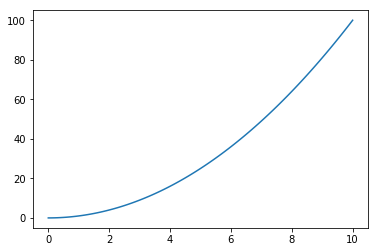Great! From here we could add more plots, linestyles, x labels, y labels, titles and more using various methods:

In :
plt.plot(x,x**2,x,x**3)
plt.xlabel("x")
plt.ylabel("y")
plt.title("Graph of x squared and x cubed")

Out:
Text(0.5,1,'Graph of x squared and x cubed')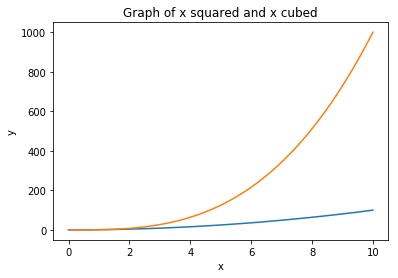We can also create multiple plots on one output. These are called subplots, and to do this we use the plt.subplot() function - this takes 3 arguments - the number of rows, the number of columns, and then the plot number we're refering to. This may seem like an ugly way to do things, and we'll see a better way to do it later with the object orientated method.

In :
plt.subplot(1,2,1)
plt.plot(x,x**2)

plt.subplot(1,2,2)
plt.plot(x**2,x,'g')

Out:
[<matplotlib.lines.Line2D at 0x1554bfc94a8>]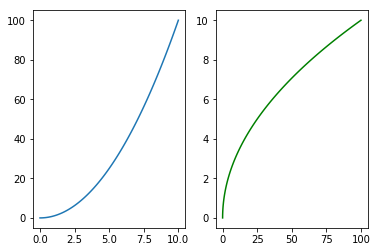## Object Orientated - There has to be a better way!¶

The problem with the functional method is that it's a bit of a mess: Commands can be a bit hard to work with and understand at a glance, and problems often happen because of ambiguity in code. Python works on an "object orientetated" philosophy so it makes sense for us to use a similar methodology for graphing. The object orientated way of creating plots works by creating figure objects and calling methods on it.

To start, we need to create a figure:

In :
fig = plt.figure()

<matplotlib.figure.Figure at 0x1554bbf1f98>

Figures are a blank canvas for us to work on. To add axis, we use the add.axes() method. We can always look at our figure by just running it as a line of code:

In :
axes = fig.add_axes([0,0,1,1])

fig

Out: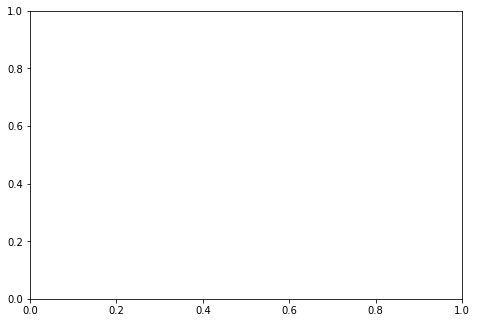Techinically, the "add_axes" method takes in one arguement - a list. This list has 4 elements - the x position with respect to the left of the axis (in percent), the y position with respect to the bottom of the axis (also in percent) - so here, 0,0 just means we don't want white space - and width and height (so here, 1,1 means width 1, height 1).

Now if we want to plot something on these axis, we call the "plot" method with respect to the axes:

In :
axes.plot(x,y)

fig

Out: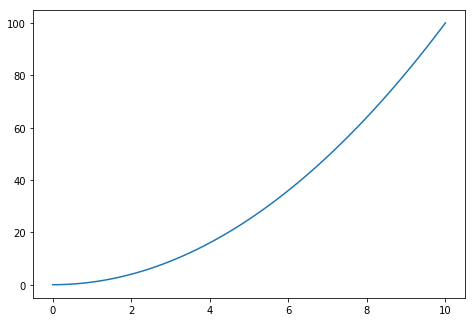We can also add x labels, y lables, and more using the following methods:

In :
axes.set_xlabel('X')
axes.set_ylabel('Y')
axes.set_title('x vs x squared')

fig

Out: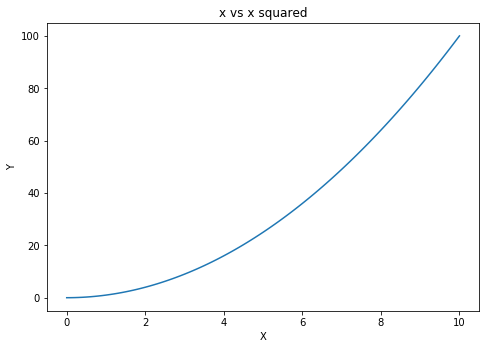Now let's see why the object orientated way of creating graphs is so much more powerful:

To start, we can create graphs within graphs:

In :
fig = plt.figure()

axes2 = fig.add_axes([0.1,0.6,0.4,0.3]) #Creating axes within our axes

axes1.plot(x,y)
axes2.plot(y,x)

axes1.set_xlabel('X')
axes1.set_ylabel('Y')
axes1.set_title('x vs x squared')

axes2.set_xlabel('X')
axes2.set_ylabel('Y')
axes2.set_title('Inverse')

Out:
Text(0.5,1,'Inverse')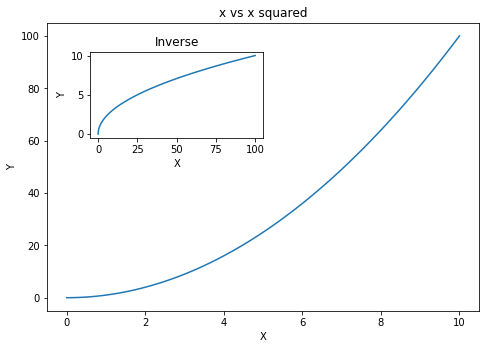We can also create subplots as before using a shortcut:

In :
fig, axes = plt.subplots()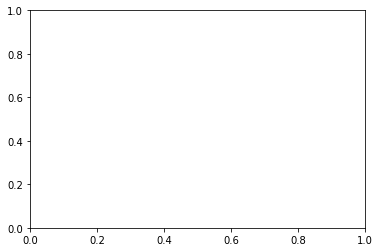This combines the "fig" and "axes" call together assuming we want the large plot by using tuple unpacking. We can add arguements to the subplot method to create more plots:

In :
fig, axes = plt.subplots(2,2)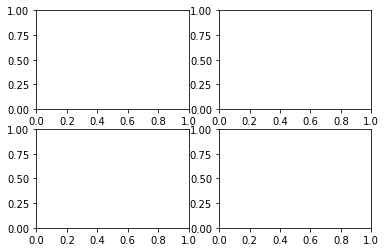Notice that all the numbers are a little bit squished together - we can fix this using the plt.tight_layout() function:

In :
fig, axes = plt.subplots(2,2)
plt.tight_layout()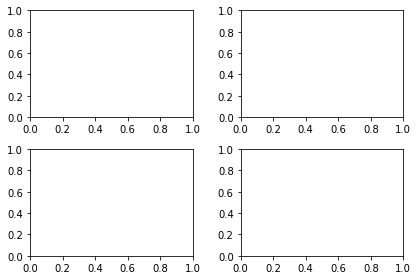Great! For now, to learn how to access these axes let's work with a 2x1 subplot grid.

If we call the "axes" object after using the subplot command, we can see that "axes" is just a list of axes objects:

In :
fig, axes = plt.subplots(1,2)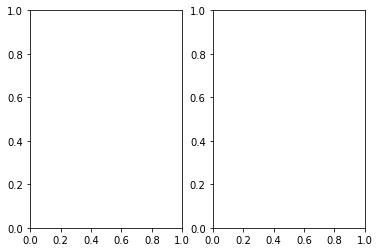In :
axes #Notice the square brackets? It's a list (technically a numpy array)!

Out:
array([<matplotlib.axes._subplots.AxesSubplot object at 0x000001554D39DA58>,
<matplotlib.axes._subplots.AxesSubplot object at 0x000001554D3E0B70>], dtype=object)

This means we can iterate over it, but more importantly, we can select what axes we want by using indexing:

In :
axes.plot(x,x)
axes.plot(x,x**2)

fig

Out: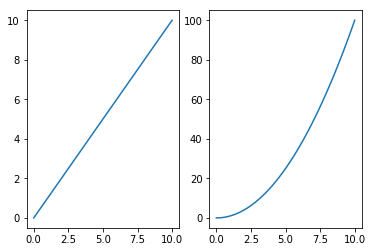Say we don't want to use two axes and we'd rather use one set of axes with two lines. We already know how to do this:

In :
fig, ax = plt.subplots()

ax.plot(x,x)
ax.plot(x,x**2)
ax.plot(x,x**3)

Out:
[<matplotlib.lines.Line2D at 0x1554d4e09b0>]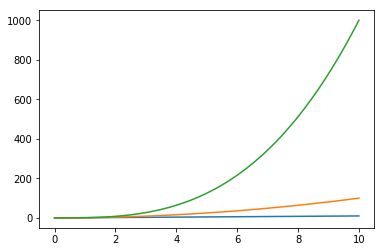How can we tell at a glance which plot is which? A legend would be helpful here - to do this we need to edit out code a little bit:

In :
fig, ax = plt.subplots()

ax.plot(x,x, label='Linear')
ax.plot(x,x**2, label='Squared')
ax.plot(x,x**3, label='Cubed')

ax.legend()

Out:
<matplotlib.legend.Legend at 0x1554d541e10>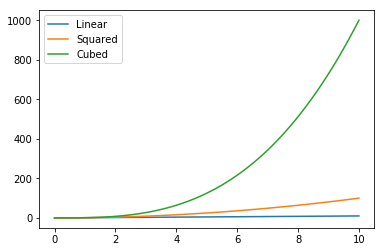Notice how we need to label our plots within their respective methods, and then call the legend method. A similar method is used to change the color and linestyles of these plots:

In :
fig, ax = plt.subplots()

ax.plot(x,x, label='Linear', color = "purple", lw=3,ls=':')
ax.plot(x,x**2, label='Squared', color = "pink", lw =3,ls='-.')
ax.plot(x,x**3, label='Cubed', color = "blue", lw=3,ls='--')

ax.legend()

Out:
<matplotlib.legend.Legend at 0x1554d5bbe10>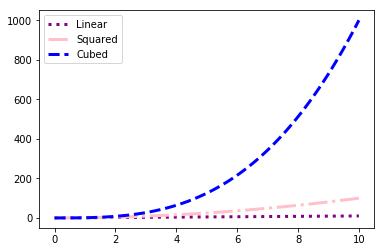There are hundreds of different plot options out there, as well as the option for 3d plots, contour plots, log scaling and more! To check these out, take a look at the documentation here

Finally, it's worth noting that we can use the object orientated method for scatter graphs, boxplots and histograms.

To start, let's take a scatter plot looking at a (albeit fake) positive correlation.

In :
fig, axes = plt.subplots()

randomData = 0.4 * np.random.randn(101) + 0.5 #Nice way to fake a correlation - 0.4 is the slope, 0.5 is the intercept.

axes.scatter(x,randomData + x)

Out:
<matplotlib.collections.PathCollection at 0x1554d62bd30>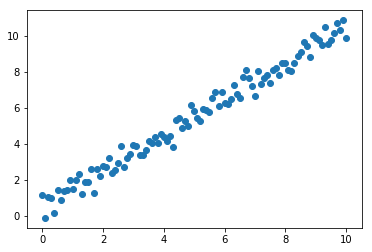Next, let's look at how to create a histogram representing a normal distribution:

In :
fig, axes = plt.subplots()

data = np.random.randn(1001)

axes.hist(data)

Out:
(array([   1.,   15.,   76.,  156.,  249.,  267.,  152.,   61.,   20.,    4.]),
array([-3.38632307, -2.71377809, -2.04123311, -1.36868813, -0.69614314,
-0.02359816,  0.64894682,  1.3214918 ,  1.99403678,  2.66658176,
3.33912675]),
<a list of 10 Patch objects>)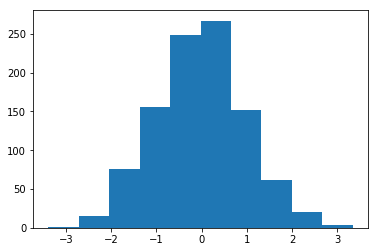And finally, let's look at boxplots:

In :
fig, axes = plt.subplots()

data = [np.random.normal(0,1,100),np.random.normal(0,2,100),np.random.normal(0,3,100)]

axes.boxplot(data)

Out:
{'boxes': [<matplotlib.lines.Line2D at 0x1554c2757f0>,
<matplotlib.lines.Line2D at 0x1554c2c3ef0>,
<matplotlib.lines.Line2D at 0x1554c2c8160>],
'caps': [<matplotlib.lines.Line2D at 0x1554c275a90>,
<matplotlib.lines.Line2D at 0x1554c2c38d0>,
<matplotlib.lines.Line2D at 0x1554c1e66d8>,
<matplotlib.lines.Line2D at 0x1554c2c8fd0>,
<matplotlib.lines.Line2D at 0x1554c29d518>,
<matplotlib.lines.Line2D at 0x1554c1ae6a0>],
'fliers': [<matplotlib.lines.Line2D at 0x1554c2c3588>,
<matplotlib.lines.Line2D at 0x1554c2c8ba8>,
<matplotlib.lines.Line2D at 0x1554c1ae748>],
'means': [],
'medians': [<matplotlib.lines.Line2D at 0x1554c2c34e0>,
<matplotlib.lines.Line2D at 0x1554c2c8e48>,
<matplotlib.lines.Line2D at 0x1554c1aefd0>],
'whiskers': [<matplotlib.lines.Line2D at 0x1554c275f28>,
<matplotlib.lines.Line2D at 0x1554c2751d0>,
<matplotlib.lines.Line2D at 0x1554c1e6470>,
<matplotlib.lines.Line2D at 0x1554c1e6c50>,
<matplotlib.lines.Line2D at 0x1554c29dcf8>,
<matplotlib.lines.Line2D at 0x1554c29d198>]}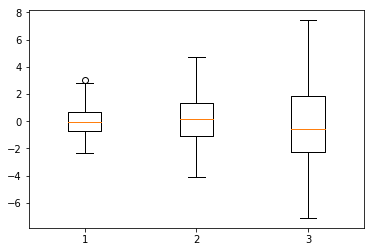Notice how the boxplot and histogram methods also output a lot of other useful information about the plots to access later if we want to. If we don't want these, we can just run fig again in another cell, or omit %matplotlib inline:

In :
fig

Out:## Worked Example¶

We're going to create a graph that looks at what happens to the graph of ex, 2x, 3x, x2 and x3. Soon we will be able to import data so we're not working the mathematical functions all the time!

In :
import matplotlib.pyplot as plt
import numpy as np
%matplotlib inline

x = np.linspace(0,10,101)

fig = plt.figure()

axes.plot(x, np.exp(x), label = "Exponential")
axes.plot(x, 2**x, label = "2 Power")
axes.plot(x, 3**x, label = "3 Power")
axes.plot(x, x**2, label = "Squared")
axes.plot(x, x**3, label = "Cubed")

axes.set_ylim((0,1000))
axes.legend()

Out:
<matplotlib.legend.Legend at 0x1554c1a08d0>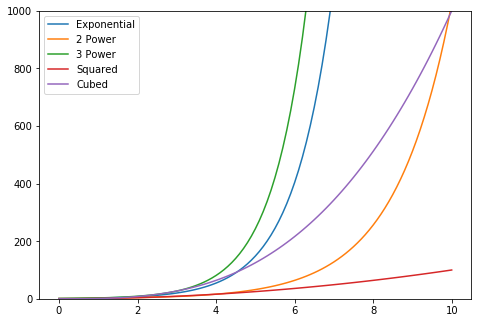## Mini Project¶

Try graphing the sin(), cos() and tan() functions on one graph, adding a legend, title and axis. Then, try using the subplot command to put them all on different plots.